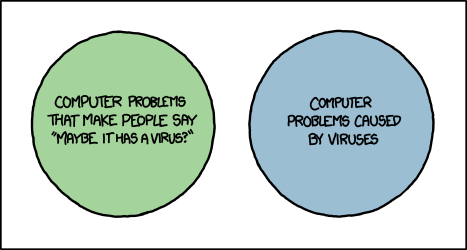# Statistics

• Posted on: 16 June 2022
Block:
Term:
Year:
LA Code:
Code:
Room:
Learning Area:
Levels:
8

Finish Probability Concepts then move on to Probability distributionsProbability Concepts
The exam is external and worth 4 credits.
You need to be familiar with methods related to:

• true probability versus model estimates versus experimental estimates (probabilities to be calculated from formulae, a probability distribution table or graph, tables of counts or proportions, simulation results)
• randomness
• independence
• mutually exclusive events
• conditional probabilities
• probability distribution tables and graphs (probabilities to be calculated from formulae, a probability distribution table or graph, tables of counts or proportions, simulation results)
• two way tables
• probability trees
• Venn diagrams.
• Assessment Opportunities: